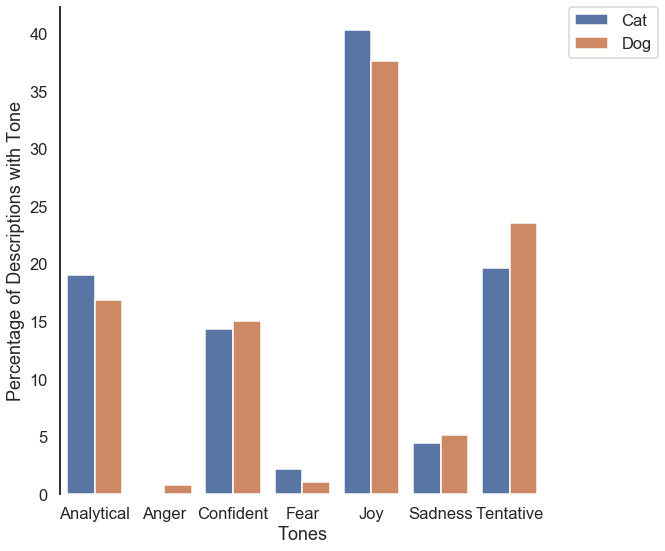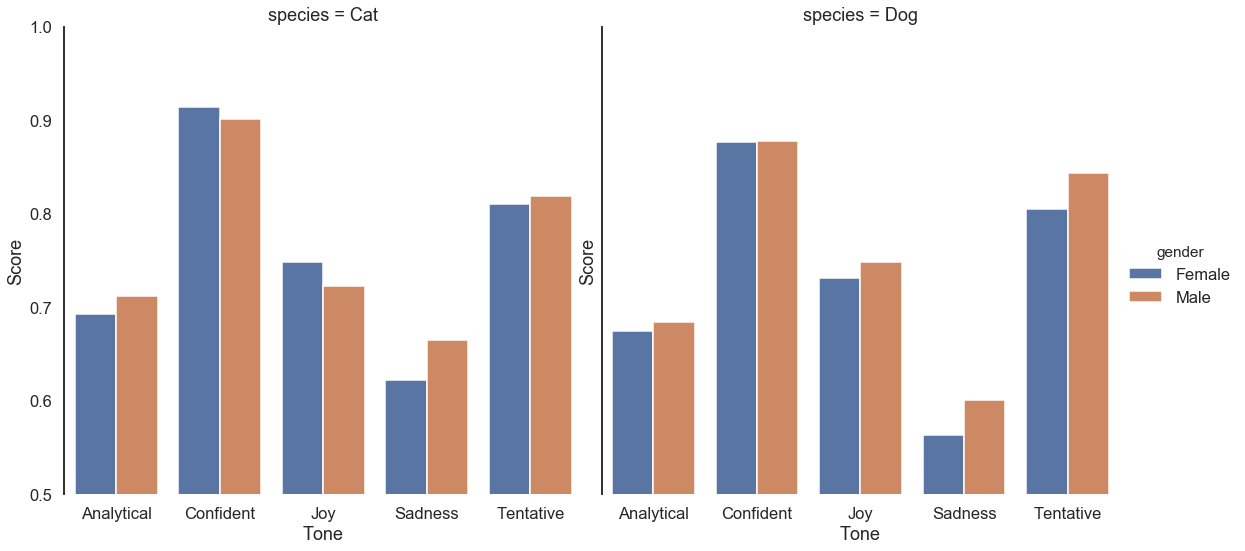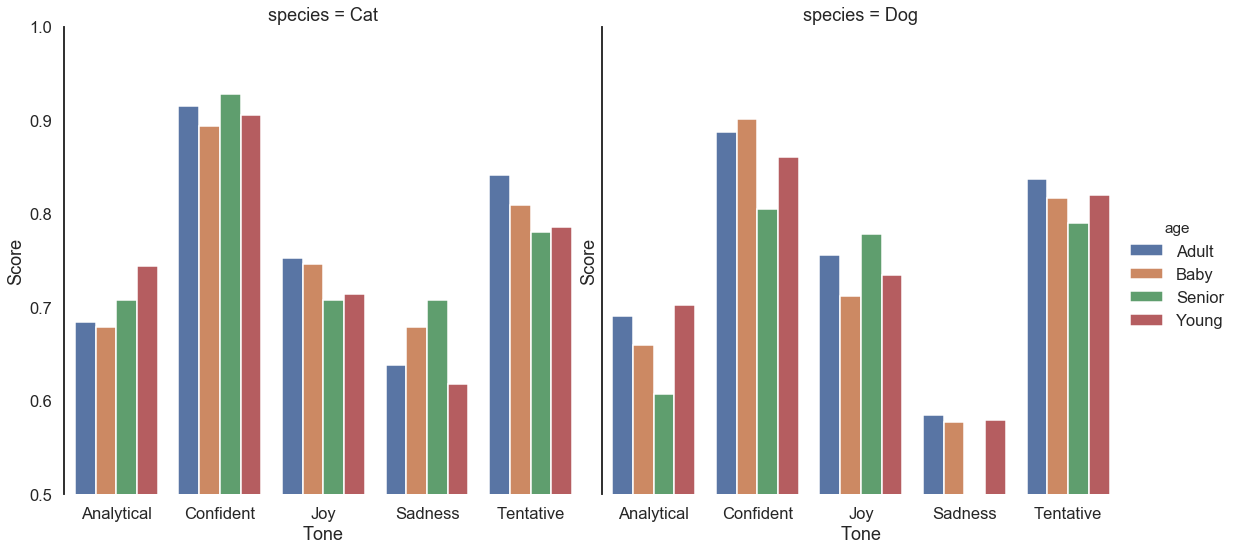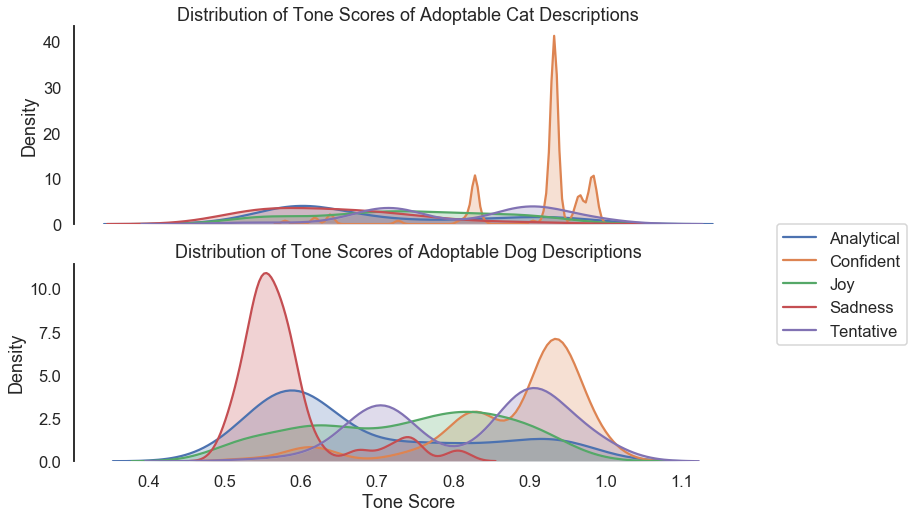## Analyzing Adoptable Pet Descriptions from Petfinder with IBM Watson Part Two

In the first part of this series, we extracted adoptable cat and dog information from Petfinder. We found the tones used in the descriptions of the adoptable animals using IBM Watson's Tone Analyzer. These datasets were then combined and cleaned to create a single, unified dataset that can be analyzed with standard Python data analysis packages.

In this post, we will explore the dataset and try to answer our original question. Is there a significant difference in tones used in adoptable animal descriptions depending on the species or other factors? For those interested in diving straight into the analysis, the extracted and cleaned datasets are available from Amazon S3.

As usual, we start by importing the libraries that will be used throughout the following analysis. Seaborn's set function is used to set the theme of the produced visualizations. We also load the dataset that was compiled in the previous post using pandas' read_csv.

In :
%matplotlib inline

import numpy as np
import pandas as pd
import seaborn as sns
import matplotlib.pyplot as plt
import scipy.stats as stats

import warnings
warnings.simplefilter('ignore')

sns.set(style="white", context="talk")



### Count of Tones Used by Species¶

Let's see if there are any significant differences in the count of tones used in adoptable cat and dog descriptions. Before plotting the data to understand the counts, we need to transform the loaded dataset into a format that can be easily read by seaborn. The pandas' function pivot_table is used to transform the dataset and aggregate the tone scores using the mean. We then create a DataFrame for the cat and dog descriptions and the count and percentage of each tone used and combine the datasets with pandas' concat.

We then display the created DataFrame to confirm it is what we need for plotting.

In :
cat_dog_pivot = pd.pivot_table(cat_dog_tones,
index=['species', 'age', 'gender', cat_dog_tones.index],
columns=['tone_name'], values=['score'],
aggfunc=np.mean).reset_index()

cat_descrip = pd.DataFrame({'count': cat_dog_pivot[cat_dog_pivot['species'] == 'Cat']['score'].count(),
'percentage': cat_dog_pivot[cat_dog_pivot['species'] == 'Cat']['score'].count() /
cat_dog_pivot[cat_dog_pivot['species'] == 'Cat'].shape * 100,
'animal': 'Cat'})

dog_descrip = pd.DataFrame({'count': cat_dog_pivot[cat_dog_pivot['species'] == 'Dog']['score'].count(),
'percentage': cat_dog_pivot[cat_dog_pivot['species'] == 'Dog']['score'].count() /
cat_dog_pivot[cat_dog_pivot['species'] == 'Dog'].shape * 100,
'animal': 'Dog'})

tone_counts = pd.concat([cat_descrip, dog_descrip]).reset_index()
tone_counts

Out:
tone_name count percentage animal
0 Analytical 158 19.013237 Cat
1 Anger 1 0.120337 Cat
2 Confident 119 14.320096 Cat
3 Fear 18 2.166065 Cat
4 Joy 335 40.312876 Cat
6 Tentative 163 19.614922 Cat
7 Analytical 127 16.865870 Dog
8 Anger 6 0.796813 Dog
9 Confident 113 15.006640 Dog
10 Fear 8 1.062417 Dog
11 Joy 283 37.583001 Dog
13 Tentative 177 23.505976 Dog

Great! With the data transformed, we can now plot the data with seaborn's catplot.

In :
b = sns.catplot(x='tone_name', y='percentage', hue='animal', kind='bar', data=tone_counts, size=8, legend=False)
b.set(xlabel='Tones', ylabel='Percentage of Descriptions with Tone')

sns.despine(bottom=True)

Out:
<matplotlib.legend.Legend at 0x1066c6ad0>The percentage of tones used in adoptable dog and cat descriptions are somewhat equal overall. What's more, Joy is the most used tone! Negative tones, such as Anger, Fear, and Sadness are the least used tones in all descriptions, which one would hope to see as these tones would likely have a downward effect on adoption rates. Analytical tones are probably those that describe the adoptable animal in detail.

We now know there are no significant differences in the percentage of tones used in adoptable cat and dog descriptions. Let's see if there is any meaningful difference in how tones are used by gender and age of the animal.

### Tones Used by Animal Age and Gender¶

As we have already transformed the data in a previous step, we can melt the data using the DataFrame melt method. The tones Fear and Anger are also removed with .loc due to their low percentage to get a clearer picture of how different factors effect the tones used in descriptions.

In :
mean_tone_scores = cat_dog_pivot.melt(id_vars=['species', 'age', 'gender'],
value_vars='score')

mean_tone_scores = mean_tone_scores.loc[(mean_tone_scores['tone_name'] != 'Fear') &
(mean_tone_scores['tone_name'] != 'Anger')]


We are now ready to plot the data! Note we are now looking at the actual mean scores of the tones as returned by IBM Watson. In contrast, before, we were investigating the raw counts of the total tones found in the descriptions.

In :
g = sns.catplot(data=mean_tone_scores, x='tone_name', y='value',
hue='gender', col='species', kind='bar', size=8,
col_wrap=2, ci=None)

g.set(ylim=(0.5,1), xlabel='Tone', ylabel='Score')
sns.despine(bottom=True)The mean score of each tone used does not appear to differ significantly by animal or gender. However, the Sadness tone has a higher mean score in cats compared to dogs. Let's now investigate if the tone scores by animal age appear to be different. The Petfinder API returns four categories Baby, Young, Adult, and Senior to describe the age of an animal.

In :
g = sns.catplot(data=mean_tone_scores, x='tone_name', y='value',
hue='age', col='species', kind='bar', size=8,
col_wrap=2, ci=None, sharey=True)
g.set(ylim=(0.5,1), xlabel='Tone', ylabel='Score')
sns.despine(bottom=True)Somewhat similarly to the average tone score by gender, the tone by animal's age does not seem to be meaningfully different within the particular species. However, the Analytical tone score appears to comparatively low for senior dogs compared to other age groups. However, we do see some differences when comparing age groups between dogs and cats. Sadness has a much higher average score across all cat age groups, while Joy scores appear to be somewhat lower in most cat age groups compared to dogs. This difference is also what we noticed when investigating the animal's gender, which gives us evidence these two tones may be significantly different between animal species.

### Testing for Statistically Significant Differences¶

Let's be more scientific and employ statistical testing methods for comparing more than two groups. Before going straight into testing for differences, it is recommended to first investigate the distributions of the groups to see if they are normally distributed. Many commonly used statistical tests such as Analysis of Variance, t-tests and others assume the sample groups have a normal distribution. If this assumption does not hold, the results of an analysis of variance procedure or other tests can produce incorrect results.

To visualize the distributions of each tone score for dogs and cats, we can use seaborn's kdeplot function, which fits and plots a univariate (or bivariate) kernel density estimate. A kernel density estimation is a nonparametric approach to estimate the probability distribution function of a variable.

In :
f, ax = plt.subplots(2, 1, figsize=(12, 8), sharex=True)

tones = ['Analytical', 'Confident', 'Joy', 'Sadness', 'Tentative']

for t in tones:
k = sns.kdeplot(cat_dog_pivot[cat_dog_pivot['species'] == 'Cat']['score'][t],
k1 = sns.kdeplot(cat_dog_pivot[cat_dog_pivot['species'] == 'Dog']['score'][t],

k.set(title='Distribution of Tone Scores of Adoptable Cat Descriptions',
ylabel='Density')

k1.set(title='Distribution of Tone Scores of Adoptable Dog Descriptions',
ylabel='Density', xlabel='Tone Score')

sns.despine(bottom=True)


Out:
<matplotlib.legend.Legend at 0x1227fdfd0>The estimated probability distribution functions of the tone scores for both cats and dogs do not appear to be normally distributed, due to the multiple peaks and long tails that can be seen for most score distributions. Now that we know the data we are analyzing is not normally distributed, we can turn to a nonparametric statistical analysis method that is robust to departures from normality. A commonly used and powerful test is the Kruskal-Wallis one-way analysis of variance test, which can be thought of as a nonparametric one-way analysis of variance procedure. Nonparametric methods do not assume the data is normally distributed and are, therefore, perfect for our current setting.

The scipy library has a kruskal function for performing the Kruskal-Wallis test on two or more samples. Using the pivoted data, we can loop through the tone score columns while filtering the data by species.

In :
for i in cat_dog_pivot['score'].columns:
print(i)
print(stats.kruskal(cat_dog_pivot[cat_dog_pivot['species'] == 'Cat']['score'][i],
cat_dog_pivot[cat_dog_pivot['species'] == 'Dog']['score'][i], nan_policy='omit'),
'\n')

Analytical
KruskalResult(statistic=3.5599861906608594, pvalue=0.05918823128270772)

Anger
KruskalResult(statistic=0.2692307692307692, pvalue=0.6038482460673433)

Confident
KruskalResult(statistic=11.208950944333806, pvalue=0.0008140372514059772)

Fear
KruskalResult(statistic=0.11149228130360522, pvalue=0.7384515491949779)

Joy
KruskalResult(statistic=0.16088487465924875, pvalue=0.6883431376465514)

KruskalResult(statistic=8.024290267683599, pvalue=0.004615410852717422)

Tentative
KruskalResult(statistic=0.04205990884909919, pvalue=0.8375056172878572)



The Kruskal-Wallis test reported a significant difference in the Sadness and Confident tones in adoptable cat and dog descriptions, given by a p-value less than 0.05. The Analytical tone was just over 0.05, so this tone could be significantly different with additional samples. These results confirm our suspicions that the Sadness and Confident tones were different in adoptable cat and dog descriptions when visualizing the data earlier.

### Conclusion¶

In this analysis, we investigated if there were any significant differences between the tones used in describing adoptable cats and dogs on Petfinder. According to our results, we can say that the tones in descriptions of both animals are not similar in that a few tones are different between the adoptable animal descriptions. Some other interesting findings include:

• The Sadness tone has a much higher mean score in adoptable cat descriptions compared to dogs. Still, at the same time, cat descriptions have a stronger tone of confidence, especially in senior cats.
• The gender of the animal does not appear to have any meaningful difference in the tone used in descriptions as the mean scores were somewhat equal across animal gender.
• Fear and Anger tones were not found in most descriptions, regardless of species or other factors.